# Python | Pandas Series.ptp()

Pandas series is a One-dimensional ndarray with axis labels. The labels need not be unique but must be a hashable type. The object supports both integer- and label-based indexing and provides a host of methods for performing operations involving the index.

Pandas` Series.ptp()` function returns the difference between the maximum value and the
minimum value in the object. This is the equivalent of the `numpy.ndarray` method `ptp`.

Syntax: Series.ptp(axis=None, skipna=None, level=None, numeric_only=None, **kwargs)

Parameter :
axis : Axis for the function to be applied on.
skipna : Exclude NA/null values when computing the result.
level : If the axis is a MultiIndex (hierarchical), count along a particular level, collapsing into a scalar.
numeric_only : Include only float, int, boolean columns. If None, will attempt to use everything, then use only numeric data. Not implemented for Series.
**kwargs : Additional keyword arguments to be passed to the function.

Returns : ptp : scalar or Series (if level specified)

Example #1: Use `Series.ptp()` function to return the difference between the maximum and the minimum value of the underlying data in the given Series object.

 `# importing pandas as pd ` `import` `pandas as pd ` ` `  `# Creating the Series ` `sr ``=` `pd.Series([``10``, ``25``, ``3``, ``11``, ``24``, ``6``]) ` ` `  `# Create the Index ` `index_ ``=` `[``'Coca Cola'``, ``'Sprite'``, ``'Coke'``, ``'Fanta'``, ``'Dew'``, ``'ThumbsUp'``] ` ` `  `# set the index ` `sr.index ``=` `index_ ` ` `  `# Print the series ` `print``(sr) `

Output :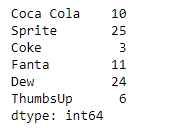Now we will use `Series.ptp()` function to find the difference between the max and min value in the given series object.

 `# return the difference between the  ` `# maximum and the minimum value ` `result ``=` `sr.ptp() ` ` `  `# Print the result ` `print``(result) `

Output :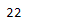As we can see in the output, the `Series.ptp()` function has successfully returned the difference between the maximum and the minimum value of the underlying data in the given series object.

Example #2: Use `Series.ptp()` function to return the difference between the maximum and the minimum value of the underlying data in the given Series object.

 `# importing pandas as pd ` `import` `pandas as pd ` ` `  `# Creating the Series ` `sr ``=` `pd.Series([``11``, ``21``, ``8``, ``18``, ``65``, ``84``, ``32``, ``10``, ``5``, ``24``, ``32``]) ` ` `  `# Print the series ` `print``(sr) `

Output :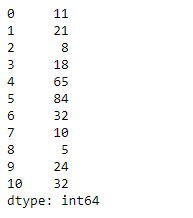Now we will use `Series.ptp()` function to find the difference between the max and min value in the given series object.

 `# return the difference between the  ` `# maximum and the minimum value ` `result ``=` `sr.ptp() ` ` `  `# Print the result ` `print``(result) `

Output :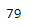As we can see in the output, the `Series.ptp()` function has successfully returned the difference between the maximum and the minimum value of the underlying data in the given series object.

Example #3: Use `Series.ptp()` function to return the difference between the maximum and the minimum value of the underlying data in the given Series object. The given series object contains some missing values in it.

 `# importing pandas as pd ` `import` `pandas as pd ` ` `  `# Creating the Series ` `sr ``=` `pd.Series([``19.5``, ``16.8``, ``None``, ``22.78``, ``None``, ``20.124``, ``None``, ``18.1002``, ``None``]) ` ` `  `# Print the series ` `print``(sr) `

Output :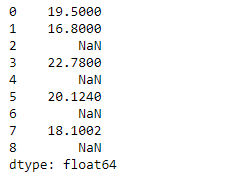Now we will use `Series.ptp()` function to find the difference between the max and min value in the given series object. we are going to skip the missing values in the calculation.

 `# return the difference between the  ` `# maximum and the minimum value ` `result ``=` `sr.ptp(skipna ``=` `True``) ` ` `  `# Print the result ` `print``(result) `

Output :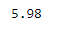As we can see in the output, the `Series.ptp()` function has successfully returned the difference between the maximum and the minimum value of the underlying data in the given series object.

My Personal Notes arrow_drop_upCheck out this Author's contributed articles.

If you like GeeksforGeeks and would like to contribute, you can also write an article using contribute.geeksforgeeks.org or mail your article to contribute@geeksforgeeks.org. See your article appearing on the GeeksforGeeks main page and help other Geeks.

Please Improve this article if you find anything incorrect by clicking on the "Improve Article" button below.

Article Tags :

Be the First to upvote.

Please write to us at contribute@geeksforgeeks.org to report any issue with the above content.